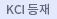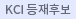#다국어 입력

http://chineseinput.net/에서 pinyin(병음)방식으로 중국어를 변환할 수 있습니다.

변환된 중국어를 복사하여 사용하시면 됩니다.

예시)
• 中文 을 입력하시려면 zhongwen을 입력하시고 space를누르시면됩니다.
• 北京 을 입력하시려면 beijing을 입력하시고 space를 누르시면 됩니다.
닫기

검색결과 좁혀 보기

• 좁혀본 항목 보기순서

• 원문유무
• 음성지원유무
• 원문제공처
• 등재정보
• 학술지명
• 주제분류
• 발행연도
• 작성언어
• 저자

오늘 본 자료

• 오늘 본 자료가 없습니다.
더보기
• 무료
• 기관 내 무료
• 유료
• Inorganic nanotube composites based on polyaniline: Potential room-temperature hydrogen storage materials

Inorganic nanotubes as a support material for polyaniline were used for hydrogen storage. To this end, a solid-state preparation method has been developed for controlling the hydrogen storage capacity of these nanocomposites. The hydrogen storage capacities have measured at room temperature and at a low pressure of 0.5 MPa using the precise quadrupole quartz crystal microbalance technique in a chamber free of moisture. The optimum nanocomposite shows an enhanced hydrogen storage capacity of 0.78 wt.% with excellent reversibility when compared to less than 0.019 wt.% capacity of the pristine nanotubes and 0.05 wt.% of polyaniline. This large increase in the hydrogen capacity can be attributed to the chemisorption hydrogen uptake, which was enhanced by the sorption sites created through the milling process of polyaniline with the nanotubes. This is in addition to the hydrogen adsorption contribution by a controlled lumen size that is suitable for a maximum hydrogen adsorption through inserting polyaniline chains into the nanotubes.

•Kinematic Analysis of the Multi-Link Five-Point Suspension System in Point Coordinates

In this paper, a numerical algorithm for the kinematic analysis of a multi-link five-point suspension system is presented. The kinematic analysis is carried out in terms of the rectangular Cartesian coordinates of some defined points in the links and at the joints. Geometric constraints are introduced to fix the relative positions between the points belonging to the same rigid body. Position, velocity and acceleration analyses are carried out. The presented formulation in terms or this system of coordinates is simple and involves only elementary mathematics. The results of the kinematic analysis are presented and discussed.

•The Effect of Suction and Injection on Unsteady Flow of a Dusty Conducting Fluid in Rectangular Channel

In the present study, the unsteady Hartmann flow of a dusty viscous incompressible electrically conducting fluid under the influence of an exponentially decreasing pressure gradient is studied without neglecting the ion slip. The parallel plates are assumed to be porous and subjected to a uniform suction from above and injection from below. The fluid is acted upon by an external uniform magnetic field which is applied perpendicular to the plates. An analytical solution for the governing equations of motion is obtained to yield the velocity distributions for both the fluid and dust particles.

•Hall Effect on Unsteady Couette Flow. with Heat Transfer Under Exponential Decaying Pressure Gradient

The unsteady Couette flow of an electrically conducting, V1SCOUS, incompressible fluid bounded by two parallel non-conducting porous plates is studied with heat transfer taking the Hall effect into consideration. An external uniform magnetic field and a uniform suction and injection are applied perpendicular to the plates while the fluid motion is subjected to an exponential decaying pressure gradient. The two plates are kept at different but constant temperatures while the Joule and viscous dissipations are included in the energy equation. The effect of the ion slip and the uniform suction and injection on both the velocity and temperature distributions is examined.

•RELATIVE MULTIFRACTAL SPECTRUM

We obtain a relation between generalized Hausdorff and packing multifractal premeasures and generalized Hausdorff and packing multifractal measures. As an application, we study a general formalism for the multifractal analysis of one probability measure with respect to an other.

•Dynamic Analysis of a Chain of Rigid Rods

In this study, a recursive algorithm for generating the equations of motion of a chain of rigid rods is presented. The methods rests upon the idea of replacing the rigid body by a dynamically equivalent constrained system of particles. The concepts of linear and angular momentums are used to generate the rigid body equations of motion without either introducing any rotational coordinates or the corresponding transformation matrices. For open-chain, the equations of motion are generated recursively along the serial chains. For closed-chain, the system is transformed to open-chain by cutting suitable kinematic joints with the addition of cut-joints kinematic constraints. An example of a closed-chain of rigid rods is chosen to demonstrate the generality and simplicity of the proposed method.

•A refined four variable plate theory for thermoelastic analysis of FGM plates resting on variable elastic foundations

In this paper, an efficient higher-order shear deformation theory is presented to analyze thermomechanical bending of temperature-dependent functionally graded (FG) plates resting on an elastic foundation. Further simplifying supposition are made to the conventional HSDT so that the number of unknowns is reduced, significantly facilitating engineering analysis. These theory account for hyperbolic distributions of the transverse shear strains and satisfy the zero traction boundary conditions on the surfaces of the plate without using shear correction factors. Power law material properties and linear steady-state thermal loads are assumed to be graded along the thickness. Nonlinear thermal conditions are imposed at the upper and lower surface for simply supported FG plates. Equations of motion are derived from the principle of virtual displacements. Analytical solutions for the thermomechanical bending analysis are obtained based on Fourier series that satisfy the boundary conditions (Navier's method). Non-dimensional results are compared for temperature-dependent FG plates and validated with those of other shear deformation theories. Numerical investigation is conducted to show the effect of material composition, plate geometry, and temperature field on the thermomechanical bending characteristics. It can be concluded that the present theory is not only accurate but also simple in predicting the thermomechanical bending responses of temperature-dependent FG plates.

•EFFECT OF POROSITY ON THE TRANSIENT MHD GENERALIZED COUETTE FLOW WITH HEAT TRANSFER IN THE PRESENCE OF HEAT SOURCE AND UNIFORM SUCTION AND INJECTION

The transient magnetohydrodynamic (MHD) generalized Couette flow with heat transfer through a porous medium of an electrically conducting, viscous, incompressible fluid bounded by two parallel insulating porous plates is studied in the presence of uniform suction and injection and a heat source considering the Hall effect. A uniform and constant pressure gradient is imposed in the axial direction and an externally applied uniform magnetic field as well as a uniform suction and injection are applied in the direction perpendicular to the plates. The two plates are kept at different but constant temperatures while the Joule and viscous dissipations are included in the energy equation. The effect of the Hall current, the porosity of the medium and the uniform suction and injection on both the velocity and temperature distributions is investigated.

•Ion Slip Effect on the Flow Due to a Rotating Disk with Heat Transfer

The steady hydromagnetic flow due to a rotating disk is studied with heat transfer considering the ion slip. The governing equations are solved numerically using finite differences. The results show that the inclusion of the ion slip has important effects on the velocity distribution as well as the heat transfer.

•Chitosan as a Flocculant: An Approach to Improve its Solubility for Efficient Harvesting of Microalgae

Chitosan is a promising flocculant for microalgae harvesting, but its scale-up application is not economically supported yet. Low solubility of chitosan in microalgae suspension demands high dosage (as a flocculant) to destabilize the cells, and thus, increases the cost of microalgae harvesting. This study identifies efficient solvents for the chitosan, and optimizes the concentration of solvents and chitosan dose to improve the harvesting efficiency. Chitosan was dissolved in different acids, and subsequently used as a flocculant. The flocculant efficacy was measured in terms of harvesting efficiency and reduction in chemical oxygen demand (COD) of the microalgae suspension. It was found that chitosan dissolved in 0.05 M HCl showed the highest harvesting efficiency (89 ± 0.87%) at only 30 mg/L of dosage. In comparison, 270 mg/L of FeCl<sub>3</sub>·6H<sub>2</sub>O was required to attain 86 ± 0.083% of the harvesting efficiency. H<sub>2</sub>SO<sub>4</sub> dissolved chitosan required high flocculant dose (150 mg/L) and resulted in relatively low harvesting efficiency (77±0.11%). It was concluded that the efficacy of chitosan is solvent dependent, and the selection of proper solvent can decrease the dosage requirement for microalgae harvesting.